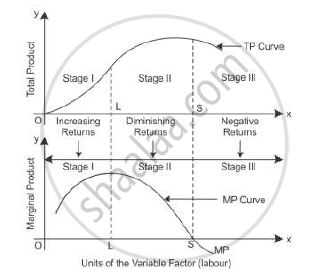# State the Behaviour of Marginal Product in the Law of Variable Proportions. Explain the Causes of this Behaviour - Economics

State the behaviour of marginal product in the law of variable proportions. Explain the causes of this behaviour

Explain the Law of Variables Proportions with the help of total product and marginal product curves.

What does the Law of Variable Proportions show? State the behaviour of the total product
according to this law

State different phases of the law of variable proportions on the basis of total product. Use Diagram.

What are the different phases of the Law of Variable Proportions in terms of marginal product? Give reason behind each phase. Use diagram

#### Solution

Law of variable proportion:-

Law of variable proportion states that as more of the variable factor input is combined with the fixed factor input, a point will eventually be reached where the marginal product of the variable factor input starts declining

 Units ofFixedFactor Units ofVariableFactor TP MP Stages 1 1 4 4 Increasing MP(Increasing returnsto a factor) 1 2 12 8 1 3 24 12 1 4 32 8 Diminishing MP (Diminishingreturns to a factor) 1 5 34 2 1 6 34 0 1 7 30 -4 Negative MP (Negative returnsto a factor) 1 8 21 -9 1 9 10 -11

Let us consider the above table.

Stage I: As more units of factor input are used, MP tends to rise till 3 units of factor input are used. Here, the total product increases at an increasing rate which is called increasing returns to the factor input.

Stage II: However, when the 4th unit of factor input is used, the diminishing returns sets in, where MP starts decreasing and TP increases at a decreasing rate. Diminishing MP reduces to zero. The total output is the maximum when the marginal output is zero.

Stage III: When MP is negative, TP starts declining from 34 to 10 when the 9th unit is employed.Concept: Law of Variable Proportions
Is there an error in this question or solution?
2011-2012 (March) Delhi Set 1
Share